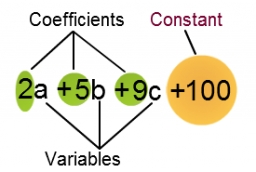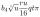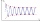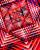# Expression with brackets

Evaluate the expression.

15+[(9-4)×22]

x =  125

### Step-by-step explanation:Did you find an error or inaccuracy? Feel free to write us. Thank you!## Related math problems and questions:

• Evaluate 9Evaluate expression: 3 { 4 − 4 ( 5 − 12 ) } + 20
• Evaluate expressionEvaluate following expression: 5 to the power of 2 - (4x4) divided by 2 =
• Evaluate expression 2Evaluate expression with negatives: (-3)+4+(-8)+(-6)+4+(-1)
• Simplify:Simplify: 8(−9 − 5x) + 4b use x = 9 and b = −9
• Evaluate expr with 2 varsIf a=6 and x=2, find the value of (2ax + 7x- 10) /(4ax-3a-2)
• BracketsPlace one pair of brackets in expression 20-16 + 10 - 4 - 2 so that the result will be 0.
• Evaluate 12Evaluate and simplify 9 divided by 7/6.
• Third dimensionCalculate the third dimension of the cuboid: a) V = 224 m3, a = 7 m, b = 4 m b) V = 216 dm3, a = 9 dm, c = 4 dm
• ExpressionsExpression (3a-7).(3a+7) is identical with: ?
• Simple equation 324 = n • 27, solve for n
• Perimeter to areaCalculate the area of a circle with a perimeter of 15 meters.
• Cube basicsHow long is the edge length of a cube with volume 15 m3?
• Expression 6Evaluate expression: -6-2(4-8)-9
• Sum of four numbersThe sum of four consecutive natural numbers is 114. Find them.
• Find unknown 2Find unknown denominator: 2/3 -5/? = 1/4
• Simple sequenceContinue with this series of numbers: 1792,448, 112, _, _
• Evaluate expressionEvaluate expression using BODMAS rule: 1 1/4+1 1/5÷3/5-5/8